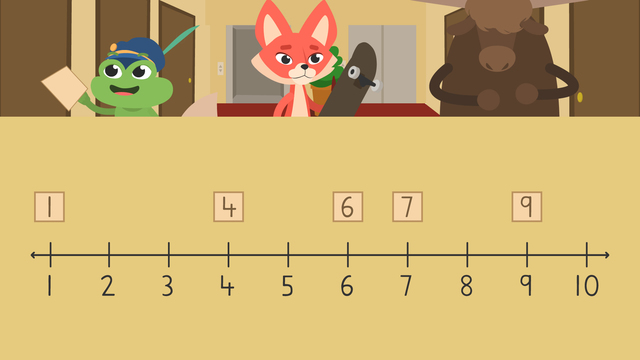# Ordering Numbers Up to 20Rating

Ø 5.0 / 1 ratings

The authorsTeam Digital
Ordering Numbers Up to 20
CCSS.MATH.CONTENT.1.NBT.A.1

## Basics on the topicOrdering Numbers Up to 20

### In This Ordering Numbers Video for 1st Grade

Henry and Skylar are visiting their friend who lives in Yosemite Tower. However, there is a problem! A buffalo moved in and every time he walks down the hall, all the numbers fall off on the doors! Now all the apartments are out of order. Now, Skylar and Henry cannot find the right apartment! In this video on ordering whole numbers, Henry and Skylar learn about ordering numbers 1 through 20 so that they can visit their friend.

### Ordering Numbers for Kids

Let’s learn more about comparing and ordering numbers with the following explanation. At the end, try it out with ordering numbers worksheets for 1st grade.

#### Ordering Numbers Definition

When we talk about the ordering of whole numbers, we mean listing things in a certain way. The numbers one through twenty follow a certain list when counting. It goes like this:If you see the numbers in different places, it's called out of order. It could look like this:To help us, we can try ordering numbers using a number line as a guide. Let’s start by ordering numbers 1-10. Here are five numbers that are out of order:Here are the numbers that are still in place.Start by comparing the numbers still in place to a number line below it.Then, find the first blank spot and look at the number line. The number line says two, so we need to find the number two and put it here.Now, move to the next blank spot and check the number line below. The number line below says three, that means the number three will go here.Let's look at the next blank spots and check them with the number line below. The number line shows us that five belongs here, eight belongs here and ten belongs here. So we put the numbers back in their places!Now that the numbers are all back in order, let's count them to check our work by counting! One, two, three, four, five, six, seven, eight, nine, ten! Ordering numbers is easy! You can try it yourself with the ordering numbers first grade worksheets below.You can repeat the same process for ordering numbers in 1st grade with the numbers eleven through twenty.

### Summary of Steps: Ordering Numbers 1-20

• First, put a number line below the list with missing numbers.

• Next, look at the missing spaces and compare them to the number line below.

• Then, write the correct numbers in their spots.

• Finally, count the order to check your work!

### Practice Ordering Numbers Activities for Grade 1

Have you practiced with ordering numbers worksheets for grade 1 yet? On this website, you can find 1st grade ordering numbers worksheets and ordering numbers activities.

### TranscriptOrdering Numbers Up to 20

Henry and Skylar are visiting a friend at Yosemite Tower. But, there is a problem! A buffalo moved in and every time he walks down the hall, the apartment numbers fall off the doors! The numbers are all out of order and they can't find their friend! Let's learn about "ordering numbers up to twenty" to help! When we talk about order, we mean listing things in a certain way. The numbers one through twenty follow a certain list when counting. It goes like this. If you see the numbers in different places, it's called out of order. To help us order numbers up to twenty, we can use a number line as a guide. Let's show Skylar how to use a number line to order apartments one through ten. Five apartment numbers are on the floor. But these apartments still have their numbers on their doors. Let's start by comparing the line of doors to a number line. Start by matching them up. Then, find the first blank door and look at the number line below. The number line says two, so we need to find the number two and put it here. Now, move to the next blank door and check the number line below. The number line below says three, that means the number three will go here. Let's look at the next blank doors and check them with the number line below. The number line shows us that five belongs here, eight belongs here and ten belongs here. Let's put the numbers back in their places! Now that the numbers are all back in order, let's count them to check our work! One, two, three, four, five, six, seven, eight, nine, ten! Great job, Skylar! Now let's help Henry. These are apartments eleven through twenty. Four apartments have numbers on the floor... and five still have numbers on the doors. Let's check with a number line to find where each number belongs. Start by matching them up. Then find the blank doors and look for those places on the number line below. What numbers will we need? We will need eleven, thirteen, sixteen and nineteen! Now we can put the numbers back where they belong and count to make sure the order is correct! Eleven, twelve, thirteen, fourteen, fifteen, sixteen, seventeen, eighteen, nineteen, twenty! While Henry and Skylar find their friend's apartment, let's review! Today we learned about ordering numbers through twenty by using a number line. First, put a number line below the list with missing numbers. Next, look at the missing spaces and compare them to the number line below. Then, write the correct numbers in their spots. Finally, count the order to check your work! "I'm glad we got everything sorted but this can't keep happening!" "You're right, Henry, I have an idea." "No more stomping down the hall now!"